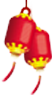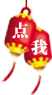# Java编程技术分享之字符串字面量

```public class ImmutableStrings
{
public static void main(String[] args)
{
String start = "Hello";
String end = start.concat(" World!");
System.out.println(end);
}
}

// Output

Hello World!```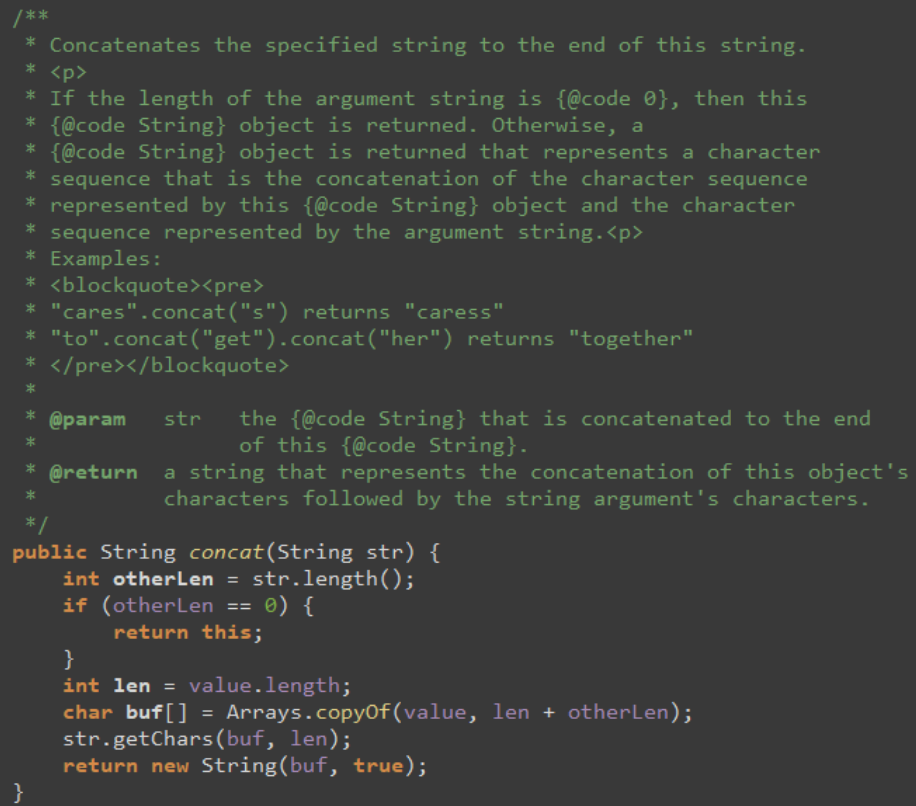```public class ImmutableStrings
{
public static void main(String[] args)
{
String one = "someString";
String two = "someString";

System.out.println(one.equals(two));
System.out.println(one == two);
}
}

// Output

true
true```

equals方法检查的是两个字符串对象是否包含相同的数据(“someString”)，而“==”操作符作用在对象上比较的是引用是否相同，这意味着只有两个引用指向的是同一个对象才会返回true。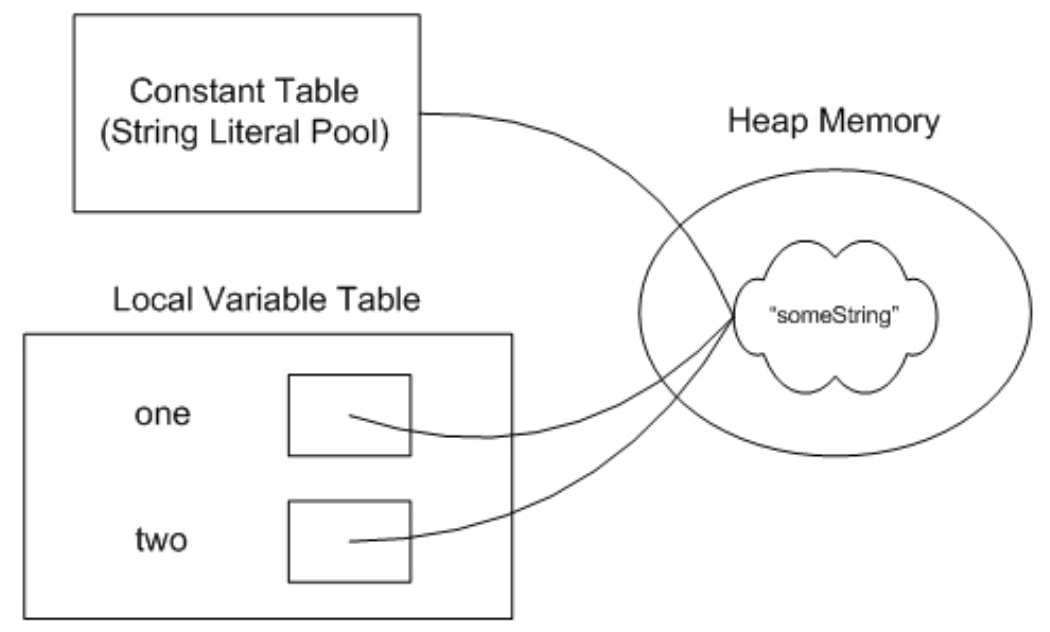```public class ImmutableStrings
{
public static void main(String[] args)
{
String one = "someString";
String two = new String("someString");

System.out.println(one.equals(two));
System.out.println(one == two);
}
}

// Output

true
false```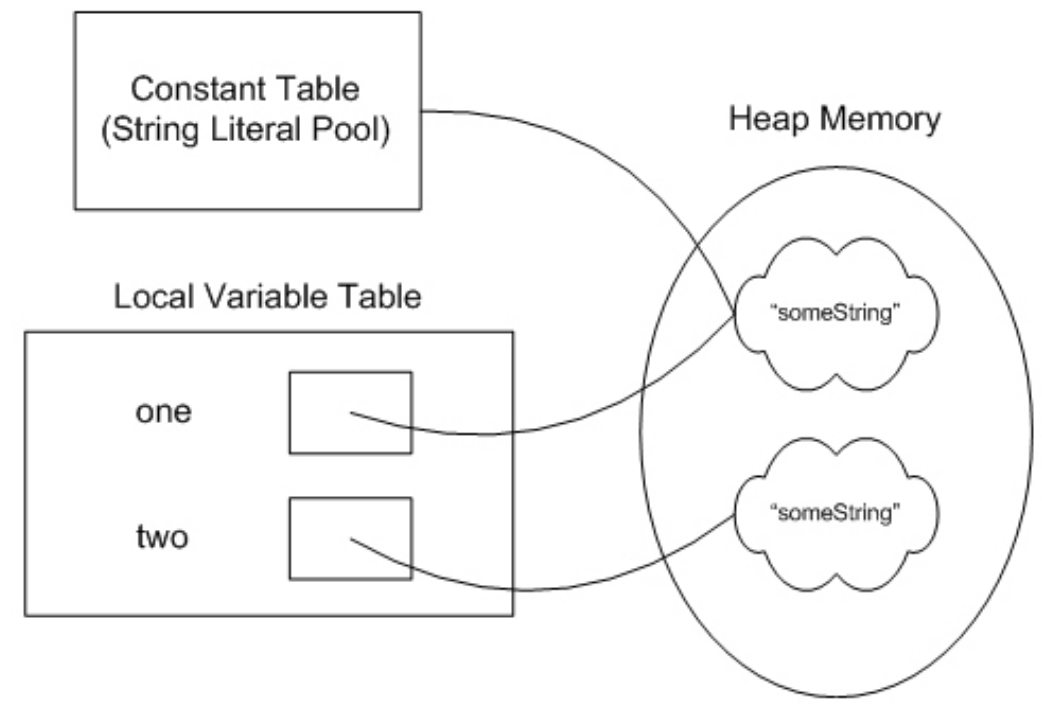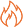HOT资讯 >

• 姓名
• 手机
• 性别
• 意向课程